2019-03-27 19:33:13 WYXHAHAHA123 阅读数 182
• ###### 第一章：机器学习

1. 介绍机器学习知识体系和学习路线 2. 机器学习的方向分析和介绍 3. 机器学习面临的核心问题，数据量，质量，特征，过拟合和欠拟合 4. 主要介绍二元分类使用，通过手写数字数据集 5. 分类问题性能考核，精度和召回，ROC曲线 6. 多分类和多标签分类器 7. 错误分析 8. MINST手写数字数据集入门案例 9. 垃圾邮件案例解析

332 人正在学习 去看看 CSDN就业班

ROC曲线是评价分类器的性能好坏的标准。

 gt\prediction 正类 负类 正类 TP FN 负类 FP TN

TP:ground truth类别为正类，prediction类别也为正类

TN:ground truth类别为负类，prediction类别也为负类

FP：ground truth类别为负类，prediction类别也为正类

FN：ground truth类别为正类，prediction类别也为负类

TPR：true positive rate =TP/(TP+FN)   预测正确的正样本总数/数据集中总正样本数

FPR：false positive rate=FP/(FP+TN)  预测错误的负样本总数/数据集中总负样本数

ROC曲线：横轴是TPR,纵轴是FPR。设定不同的判定为正负样本的阈值（threshold），可以得到不同的TPR和FPR点对。将一系列点对连接成平滑的曲线，则为ROC曲线。设想，

（1）当阈值设置为0，就是说，预测概率值大于0，则判定为正样本，小于0则判定为负样本，则对于逻辑回归分类器，所输出的预测概率值全部都大于0，故而所有的样本都会被分类为正样本，FN=TN=0，TPR=FPR=1，在ROC曲线上对应点（0，0）

（1）当阈值设置为1，就是说，预测概率值大于1，则判定为正样本，小于1则判定为负样本，则对于逻辑回归分类器，所输出的预测概率值全部都小于1，故而所有的样本都会被分类为负样本，FP=TP=0，TPR=FPR=0，在ROC曲线上对应点（1，1）

ROC曲线下包围的面积定义为AUC：areas under curve

AUC值越大，说明分类器的性能越好。

2019-04-18 10:47:44 z_feng12489 阅读数 4608
• ###### 第一章：机器学习

1. 介绍机器学习知识体系和学习路线 2. 机器学习的方向分析和介绍 3. 机器学习面临的核心问题，数据量，质量，特征，过拟合和欠拟合 4. 主要介绍二元分类使用，通过手写数字数据集 5. 分类问题性能考核，精度和召回，ROC曲线 6. 多分类和多标签分类器 7. 错误分析 8. MINST手写数字数据集入门案例 9. 垃圾邮件案例解析

332 人正在学习 去看看 CSDN就业班

# 前言简介# 医学图像识别二分类问题(1) 真阳性(True Positive，TP)：检测有结节，且实际有结节；正确肯定的匹配数目；
(2) 假阳性(False Positive，FP)：检测有结节，但实际无结节；误报，给出的匹配是不正确的；
(3) 真阴性(True Negative，TN)：检测无结节，且实际无结节；正确拒绝的非匹配数目；
(4) 假阴性(False Negative，FN)：检测无结节，但实际有结节；漏报，没有正确找到的匹配的数目。• 正确率(Precision)：
$Precision=\dfrac{TP}{TP+FP}$

• 真阳性率(True Positive Rate，TPR)，灵敏度(Sensitivity)，召回率(Recall)：
$Sensitivity=Recall=TPR=\dfrac{TP}{TP+FN}$

• 真阴性率(True Negative Rate，TNR)，特异度(Specificity)：
$Specificity=TNR=\dfrac{TN}{FP+TN}$

• 假阴性率(False Negatice Rate，FNR)，漏诊率( = 1 - 灵敏度)：
$FNR=\dfrac{FN}{TP+FN}$

• 假阳性率(False Positice Rate，FPR)，误诊率( = 1 - 特异度)：
$FPR=\dfrac{FP}{FP+TN}$

• 阳性似然比(Positive Likelihood Ratio (LR+))：
$LR+ = \dfrac{TPR}{FPR} = \dfrac{Sensitivity}{1-Specificity}$

• 阴性似然比(Negative Likelihood Ratio (LR−) )：
$LR- = \dfrac{FNR}{TNR} = \dfrac{1-Sensitivity}{Specificity}$

• Youden指数(Youden index)：
$\text{Youden index} = Sensitivity + Specificity - 1 = TPR - FPR$

# ROC 曲线• 理想情况下，TPR应该接近1，FPR应该接近0。ROC曲线上的每一个点对应于一个threshold，对于一个分类器，每个threshold下会有一个TPR和FPR。比如Threshold最大时，TP=FP=0，对应于原点；Threshold最小时，TN=FN=0，对应于右上角的点(1,1)。
• P和N得分不作为特征间距离d的一个函数，随着阈值theta增加，TP和FP都增加。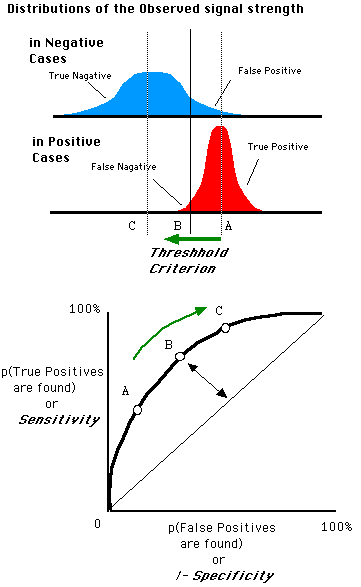# 如何画ROC曲线

In signal detection theory, a receiver operating characteristic (ROC), or simply ROC curve, is a graphical plot which illustrates the performance of a binary classifier system as its discrimination threshold is varied.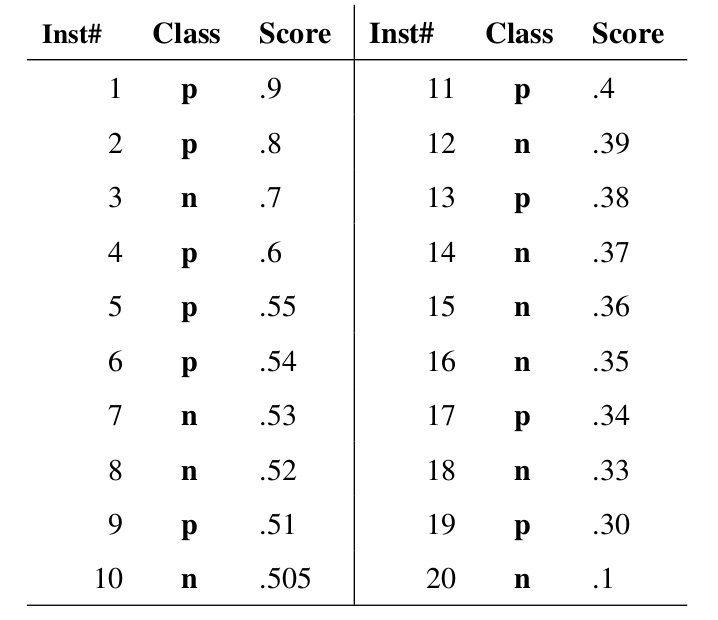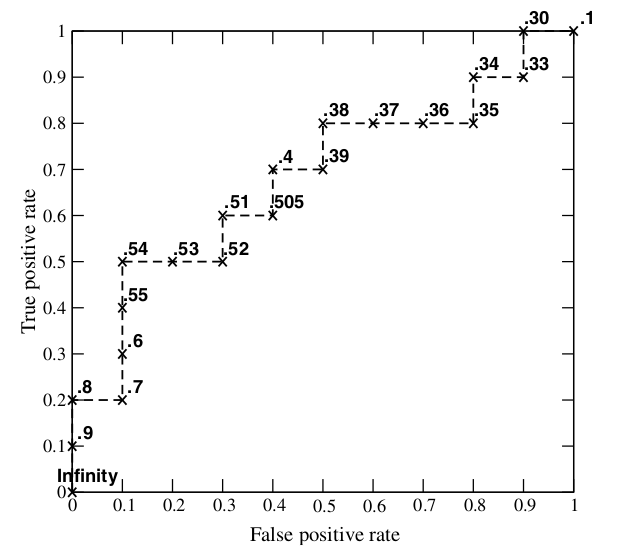其实，我们并不一定要得到每个测试样本是正样本的概率值，只要得到这个分类器对该测试样本的“评分值”即可（评分值并不一定在(0,1)区间）。评分越高，表示分类器越肯定地认为这个测试样本是正样本，而且同时使用各个评分值作为threshold。我认为将评分值转化为概率更易于理解一些。

# AUC

AUC值的计算

AUC (Area Under Curve) 被定义为ROC曲线下的面积，显然这个面积的数值不会大于1。又由于ROC曲线一般都处于y=x这条直线的上方，所以AUC的取值范围一般在0.5和1之间。使用AUC值作为评价标准是因为很多时候ROC曲线并不能清晰的说明哪个分类器的效果更好，而作为一个数值，对应AUC更大的分类器效果更好。

AUC的计算有两种方式，梯形法和ROC AUCH法，都是以逼近法求近似值，具体见wikipedia

AUC意味着什么

The AUC value is equivalent to the probability that a randomly chosen positive example is ranked higher than a randomly chosen negative example.

• AUC = 1，是完美分类器，采用这个预测模型时，存在至少一个阈值能得出完美预测。绝大多数预测的场合，不存在完美分类器。
• 0.5 < AUC < 1，优于随机猜测。这个分类器（模型）妥善设定阈值的话，能有预测价值。
• AUC = 0.5，跟随机猜测一样（例：丢铜板），模型没有预测价值。
• AUC < 0.5，比随机猜测还差；但只要总是反预测而行，就优于随机猜测。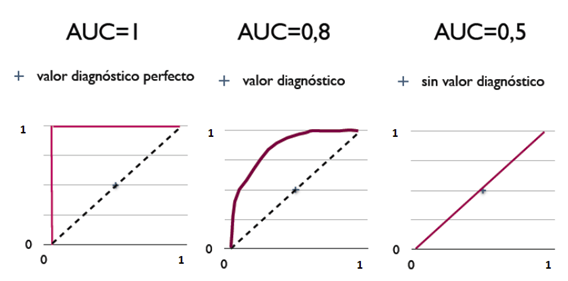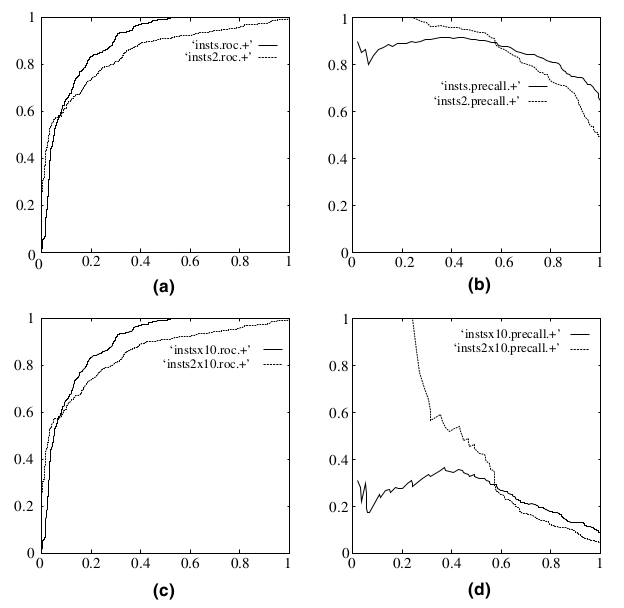Reference:

Rachel Zhang的专栏(CSDN)：ROC曲线-阈值评价标准

（在此对以上博文的博主表示感谢！）

转自 zhwhong 的博客：[机器学习之分类性能度量指标 : ROC曲线、AUC值、正确率、召回率](https://zhwhong.cn/2017/04/14/ROC-AUC-Precision-Recall-analysis/)

2017-01-13 16:46:32 taoyanqi8932 阅读数 33817
• ###### 第一章：机器学习

1. 介绍机器学习知识体系和学习路线 2. 机器学习的方向分析和介绍 3. 机器学习面临的核心问题，数据量，质量，特征，过拟合和欠拟合 4. 主要介绍二元分类使用，通过手写数字数据集 5. 分类问题性能考核，精度和召回，ROC曲线 6. 多分类和多标签分类器 7. 错误分析 8. MINST手写数字数据集入门案例 9. 垃圾邮件案例解析

332 人正在学习 去看看 CSDN就业班

## 引言

$\quad$21 Must-Know Data Science Interview Questions and Answers 的文章中，有这类似这样的问题，它问的是Explain what precision and recall are. How do they relate to the ROC curve?关于这个问题其实有许多需要回答的，不仅仅是他们的表现形式不同，同时它涉及到下机器学习中的性能度量(performance measure)问题，下面对其进行详细的说明。

## 性能度量(performance measure)

$\quad$在对学习器的泛化能力进行评估是模型泛化的能力，即要用到机器学习的性能度量，不同的性能度量往往会导致不同的评判结果，这意味着模型的好坏事相对的，什么样的模型是好的，不仅取决于算法和数据，还决定于任务的需求。
$\quad$最常见的的分类中所用的度量是：accuracy(准确度)，error rate

acc=1mi=1mI(f(xi)=yi)

err=1mi=1mI(f(xi)yi)

$\quad$上面两种度量方法非常的常用，但是有时并不能满足任务的要求，其准确度将每个类看得同等的重要，因此它可能不适合用来分析不平衡的数据集，不平衡的数据集即正类样本远远小于负类的样本，但是我们用对正类的样本比较关心，这样准确度就不适合这种度量了，因此引入了下面的几种度量方法。

$\quad$假定下面一个例子，假定在10000个样本中有100个正样本，其余为负样本，其在分类器下的混淆矩阵(confusion matrix)为：1. TN / True Negative: case was negative and predicted negative
2. TP / True Positive: case was positive and predicted positive
3. FN / False Negative: case was positive but predicted negative
4. FP / False Positive: case was negative but predicted positive

$\quad$真正率(true positive rate,TPR)或灵敏度(sensitivity)，定义为被模型正确预测的正样本的比例：

TPR=TPTP+FN

$\quad$真负率(true negative rate,TFR)或特指度(specificity)，定义为被模型正确预测的负样本的比例：

TPR=TNTN+FP

$\quad$同理，假正率(false positive rate,FPR)

FPR=FPTN+FP

$\quad$假负率(flase negative rate,FNR)
FNR=FNTP+FN

$\quad$precision(精度)，其与accuracy感觉中文翻译一致，周志华老师的书中称为：查准率:

p=TPTP+FP

$\quad$recall(召回率),周志华老师的书中称为查全率，其又与真正率一个公式：

r=TPTP+FN

$\quad$精度是确定分类器中断言为正样本的部分其实际中属于正样本的比例，精度越高则假的正例就越低，召回率则是被分类器正确预测的正样本的比例。两者是一对矛盾的度量，其可以合并成令一个度量，F1度量：

F1=2rpr+p=2TP2TP+FP+FN

$\quad$如果对于precision和recall的重视不同，则一般的形式：

Fβ=(1+β2)rpβ2p+r

$\quad$可以从公式中看到β=1$\beta=1$则退化成F1,β>1$\beta>1$则recall有更大影响，反之则precision更多影响。

$\quad$维基百科中一个非常好的关于两者之间的例子：

$\qquad\qquad\qquad\qquad$## ROC and PRC

$\quad$ROC(receiver operating characteristic)接受者操作特征，其显示的是分类器的真正率和假正率之间的关系，如下图所示：$\quad$ROC曲线有助于比较不同分类器的相对性能，当FPR小于0.36时M1浩宇M2，而大于0.36是M2较好。
$\quad$ROC曲线小猫的面积为AUC(area under curve)，其面积越大则分类的性能越好，理想的分类器auc=1。

PR(precision recall)曲线表现的是precision和recall之间的关系，如图所示：### 如何选择ROC,PR

$\quad$Particularly, if true negative is not much valuable to the problem, or negative examples are abundant. Then, PR-curve is typically more appropriate. For example, if the class is highly imbalanced and positive samples are very rare, then use PR-curve. One example may be fraud detection, where non-fraud sample may be 10000 and fraud sample may be below 100.
In other cases, ROC curve will be more helpful.

Let’s take an example of fraud detection problem where there are 100 frauds out of 2 million samples.
Algorithm 1: 90 relevant out of 100 identified
Algorithm 2: 90 relevant out of 1000 identified

Evidently, algorithm 1 is more preferable because it identified less number of false positive.
In the context of ROC curve,
Algorithm 1: TPR=90/100=0.9, FPR= 10/1,999,900=0.00000500025
Algorithm 2: TPR=90/100=0.9, FPR=910/1,999,900=0.00045502275
The FPR difference is 0.0004500225

For PR, Curve
Algorithm 1: precision=0.9, recall=0.9
Algorithm 2: Precision=90/1000=0.09, recall= 0.9
Precision difference= 0.81

### 如何绘制ROC曲线

$\quad$为了绘制ROC曲线，则分类器应该能输出连续的值，比如在逻辑回归分类器中，其以概率的形式输出，可以设定阈值大于0.5为正样本，否则为负样本。因此设置不同的阈值就可以得到不同的ROC曲线中的点。print(__doc__)

import numpy as np
from scipy import interp
import matplotlib.pyplot as plt
from itertools import cycle

from sklearn import svm, datasets
from sklearn.metrics import roc_curve, auc
from sklearn.model_selection import StratifiedKFold

###############################################################################
# Data IO and generation

# import some data to play with
X = iris.data
y = iris.target
X, y = X[y != 2], y[y != 2]
n_samples, n_features = X.shape

random_state = np.random.RandomState(0)
X = np.c_[X, random_state.randn(n_samples, 200 * n_features)]

###############################################################################
# Classification and ROC analysis

# Run classifier with cross-validation and plot ROC curves
cv = StratifiedKFold(n_splits=6)

# 注意这里的应该改为probability=True以概率形式输出
classifier = svm.SVC(kernel='linear', probability=True,
random_state=random_state)

mean_tpr = 0.0
mean_fpr = np.linspace(0, 1, 100)

colors = cycle(['cyan', 'indigo', 'seagreen', 'yellow', 'blue', 'darkorange'])
lw = 2

i = 0
# k折交叉验证
for (train, test), color in zip(cv.split(X, y), colors):
probas_ = classifier.fit(X[train], y[train]).predict_proba(X[test])
# Compute ROC curve and area the curve
#　注意这里返回的阈值，以区分正负样本的阈值
fpr, tpr, thresholds = roc_curve(y[test], probas_[:, 1])
＃　进行插值
mean_tpr += interp(mean_fpr, fpr, tpr)
mean_tpr = 0.0
roc_auc = auc(fpr, tpr)
plt.plot(fpr, tpr, lw=lw, color=color,
label='ROC fold %d (area = %0.2f)' % (i, roc_auc))

i += 1
plt.plot([0, 1], [0, 1], linestyle='--', lw=lw, color='k',
label='Luck')

mean_tpr /= cv.get_n_splits(X, y)
mean_tpr[-1] = 1.0
mean_auc = auc(mean_fpr, mean_tpr)
plt.plot(mean_fpr, mean_tpr, color='g', linestyle='--',
label='Mean ROC (area = %0.2f)' % mean_auc, lw=lw)

plt.xlim([-0.05, 1.05])
plt.ylim([-0.05, 1.05])
plt.xlabel('False Positive Rate')
plt.ylabel('True Positive Rate')
plt.legend(loc="lower right")
plt.show()１.《机器学习》周志华
２.《数据挖掘导论》
３. 21 Must-Know Data Science Interview Questions and Answers
４. What is the difference between a ROC curve and a precision-recall curve? When should I use each?
5. Receiver Operating Characteristic (ROC) with cross validation

2018-07-21 18:08:19 yqf113 阅读数 188
• ###### 第一章：机器学习

1. 介绍机器学习知识体系和学习路线 2. 机器学习的方向分析和介绍 3. 机器学习面临的核心问题，数据量，质量，特征，过拟合和欠拟合 4. 主要介绍二元分类使用，通过手写数字数据集 5. 分类问题性能考核，精度和召回，ROC曲线 6. 多分类和多标签分类器 7. 错误分析 8. MINST手写数字数据集入门案例 9. 垃圾邮件案例解析

332 人正在学习 去看看 CSDN就业班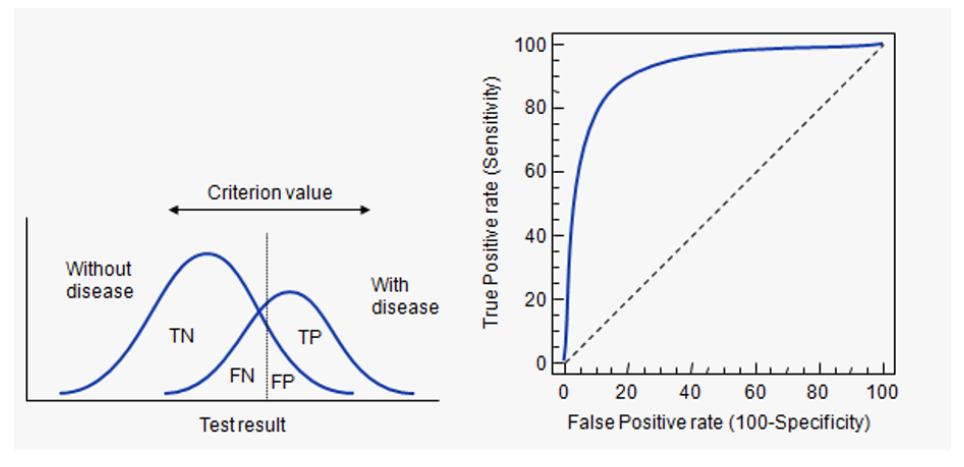PS：召回率和准确率：准确率P是TP/(TP+FP)，召回率R是TP/(TP+FN)，和真正率一样。我们希望准确率和召回率都越高越好。F1=2PR/(P+R)=2TP/(2TP+FP+FN)当FP和FN越小时，F1越接近于1.

auc和F1是两个不同的评价指标。

2019-11-06 17:01:01 CarryLvan 阅读数 53
• ###### 第一章：机器学习

1. 介绍机器学习知识体系和学习路线 2. 机器学习的方向分析和介绍 3. 机器学习面临的核心问题，数据量，质量，特征，过拟合和欠拟合 4. 主要介绍二元分类使用，通过手写数字数据集 5. 分类问题性能考核，精度和召回，ROC曲线 6. 多分类和多标签分类器 7. 错误分析 8. MINST手写数字数据集入门案例 9. 垃圾邮件案例解析

332 人正在学习 去看看 CSDN就业班

### 机器学习实战——ROC曲线和AUC值

近期在做机器学习实战上的项目时，讲解roc部分的代码没有理解，查阅相关博客资料后有所感悟理解，做下笔记记录一下。

## 1.查准率(Precision)和召回率(Recall)

通过一个例子来理解一下：我们现在需要判断一群病人中是否患有癌症。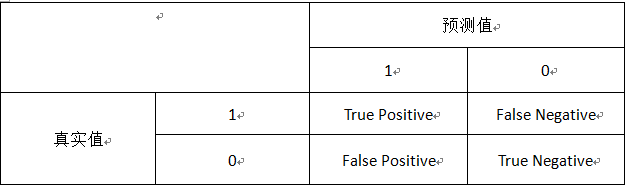上表中1表示患有癌症，0则不患有癌症。“True Positive”表示我们预测病人患有癌症，而事实是该病人确实有癌症的人数；“False Negative”表示我们预测病人没有癌症，但是事实上他却凡有癌症的人数；其他以此类推。
下面我用TP简写代表“True Positive”，其他也是。
接下来我们来看看查准率和召回率的定义：

1. 查准率(Precision) = TP/(TP+FP)
它表示的含义就是对于所有我们预测患有癌症的病人，到底有多大比例的病人是真正患有癌症的。

2. 召回率(Recall) = TP/(TP+FN)
召回率的含义是对于所有患有癌症的病人，有多少人我们可以正确的告诉他们需要治疗（即告诉他们患有癌症）。

上表也称为混淆矩阵(confusion matrix)

## 2.ROC曲线

先看到下图：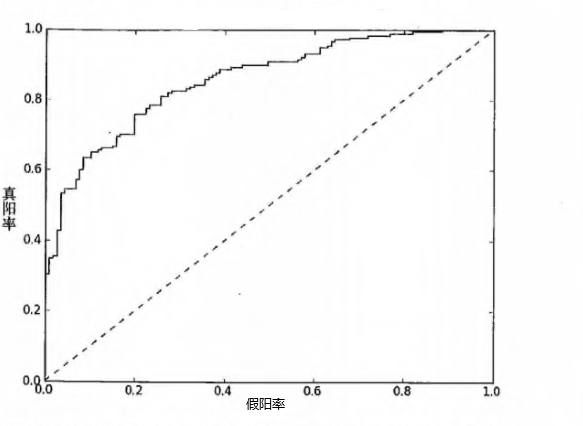横轴表示假阳率(=FP/(TN+FP))，纵轴表示真阳率(=TP/(TP+FN))。
其中假阳率可以理解为对于所有没有患癌症的病人，我们却告诉病人患有癌症的比例；真阳率就是我们的查准率。
理想情况是假阳率很低的同时有很高的真阳率：很高的真阳率即对于那些患有癌症的病人我们都告诉他们患有癌症（避免了错过治疗）；很低的假阳率即对于那些正常的病人我们正确告诉他们没有患癌症（如果错误告诉，可能会给病人带来极大的心理负担）。

如何绘制ROC曲线？
具体流程：
1.我们先通过分类器得出每个样本的预测强度（即概率大小）。
2.然后我们将所有样本按概率大小从小到大排序。
3.接着循环从最小的开始，取其值为我们的阈值，比阈值小的都预测为反例，比阈值大的预测为正例。因为我们每次都是从小到大遍历去取一个概率值作为阈值，相当于把这个样本从原来预测1变为预测0，然后结合真实值，如果真实值为1，我们以前预测为反例1现在预测0，则TP-1；如果真实值为0，我们以前预测为反例1，则FP-1

看代码：

	#cur为真阳率，cur为假阳率，初始都设为1
cur = (1.0,1.0)
ySum = 0.0
#得到总的真实值为1的正例数目
numPosClass = sum(array(classLabels)==1.0)
#步长，真阳率每次分子减一（上面就是求得总的整理的数量，因此取倒数就行），横轴同理
yStep = 1/float(numPosClass)
xStep = 1/float(len(classLabels)-numPosClass)
#predStrengths是一个向量，包含每一个样本的预测强度，这里我们从小到大排序
#argsort()返回的是排序后每个元素在数组中的下标：例. [23,24,10,56,78]得到[2 0 1 3 4]
sortedIndices = predStrengths.argsort()
#构建画笔
fig = plt.figure()
fig.clf()
ax = plt.subplot(111)
#从最小的概率值开始遍历
for index in sortedIndices.tolist():
#进行判断，如果真实值为1，在前面的循环中，也是将其预测为1，这轮循环则
#将其预测为0（因为阈值取该样本的概率值），也就是TP-1，真阳率的分子减一
if classLabels[index] == 1.0:
delX = 0; delY = yStep
else:
delX = xStep; delY = 0
ySum += cur
ax.plot([cur, cur - delX], [cur, cur - delY], c='b')
#更新当前点，即
cur = (cur - delX, cur - delY)


## 3.AUC值

AUC，Area Under the Curve，顾名思义AUC值使我们曲线线下所围成的面积。AUC给出的是分类器的平均性能值。

结合代码：
看到上面的代码，我们计算曲线围成的面积其实就是对多个小矩形进行累加，小矩形的宽度就是xStep,然后只需要将每个矩形的高度累加起来即可，只有当我们进行横轴的移动时才累加高度，看到上面因为纵轴减小时，横轴没变，此时相当于一条线，所以不需要累加。

机器学习中的roc 相关内容

阅读数 309

博文 来自： weixin_33928137

阅读数 4153

博文 来自： qq_21997625

阅读数 1139

博文 来自： daerzei

阅读数 81

博文 来自： TLP1993
没有更多推荐了，返回首页